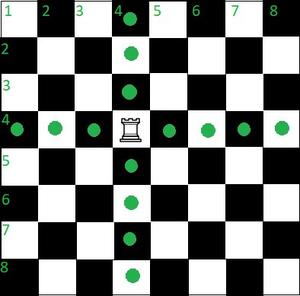Open in App
Not now

# Check if a Rook can reach the given destination in a single move

• Last Updated : 03 May, 2021

Given integers current_row and current_col, representing the current position of a Rook on an 8 × 8 chessboard and two more integers destination_row and destination_col which represents the position to be reached by a Rook. The task is to check if it is possible or not for a Rook to reach the given destination in a single move from its current position. If found to be true, print “POSSIBLE”. Otherwise, print “NOT POSSIBLE”.

Examples:

Input: current_row=8, current_col=8, destination_row=8, destination_col=4
Output: POSSIBLE
Explanation:

Input: current_row=3, current_col=2, destination_row=2, destination_col=4
Output: NOT POSSIBLE
Explanation:

Approach: The given problem can be solved using the following observation:

On a chessboard, a rook can move as many squares as possible, horizontally as well as vertically, in a single move. Therefore, it can move to any position present in the same row or the column as in its initial position.Therefore, the problem reduces to simply checking for the following two conditions:

• If destination_row and current_row are equal or not.
• Otherwise, check if destination_col is equal to current_col or not.
• If any of the above two conditions are satisfied, print “POSSIBLE“. Otherwise, print “NOT POSSIBLE”.

Below is the implementation of the above approach:

## C++14

 `// C++ program to implement` `// for the above approach` `#include ` `using` `namespace` `std;`   `// Function to check if it is` `// possible to reach destination` `// in a single move by a rook` `string check(``int` `current_row, ``int` `current_col,` `             ``int` `destination_row, ``int` `destination_col)` `{`   `    ``if``(current_row == destination_row)` `        ``return` `"POSSIBLE"``;` `    ``else` `if``(current_col == destination_col)` `        ``return` `"POSSIBLE"``;` `    ``else` `        ``return` `"NOT POSSIBLE"``;` `}`   `// Driver Code` `int` `main()` `{` `  `  `  ``// Given arrays` `  ``int` `current_row = 8;` `  ``int` `current_col = 8;` `  ``int` `destination_row = 8;` `  ``int` `destination_col = 4;` `  ``string output = check(current_row, current_col,` `                        ``destination_row, destination_col);` `  ``cout << output;` `  ``return` `0;` `}`   `// This code is contributed by mohit kumar 29.`

## Java

 `// Java program for the above approach` `import` `java.util.*; ` `import` `java.lang.*;`   `class` `GFG{ ` `    `  `// Function to check if it is` `// possible to reach destination` `// in a single move by a rook` `static` `String check(``int` `current_row, ``int` `current_col,` `             ``int` `destination_row, ``int` `destination_col)` `{`   `    ``if``(current_row == destination_row)` `        ``return` `"POSSIBLE"``;` `    ``else` `if``(current_col == destination_col)` `        ``return` `"POSSIBLE"``;` `    ``else` `        ``return` `"NOT POSSIBLE"``;` `}`   `// Driver code ` `public` `static` `void` `main(String[] args) ` `{ ` `    ``// Given arrays` `  ``int` `current_row = ``8``;` `  ``int` `current_col = ``8``;` `  ``int` `destination_row = ``8``;` `  ``int` `destination_col = ``4``;` `  ``String output = check(current_row, current_col,` `                        ``destination_row, destination_col);` `  ``System.out.println(output);` `} ` `} `   `// This code is contributed by code_hunt.`

## Python3

 `# Python program to implement` `# for the above approach`     `# Function to check if it is ` `# possible to reach destination` `# in a single move by a rook` `def` `check(current_row, current_col,` `           ``destination_row, destination_col):` `    `  `    ``if``(current_row ``=``=` `destination_row):` `        ``return``(``"POSSIBLE"``)` `    ``elif``(current_col ``=``=` `destination_col):` `        ``return``(``"POSSIBLE"``)` `    ``else``:` `        ``return``(``"NOT POSSIBLE"``)`   `# Driver Code` `current_row ``=` `8` `current_col ``=` `8` `destination_row ``=` `8` `destination_col ``=` `4`     `output ``=` `check(current_row, current_col,` `               ``destination_row, destination_col)` `print``(output)`

## C#

 `// C# program to implement` `// the above approach` `using` `System;`   `class` `GFG` `{`   `  ``// Function to check if it is` `  ``// possible to reach destination` `  ``// in a single move by a rook` `  ``static` `string` `check(``int` `current_row, ``int` `current_col,` `                      ``int` `destination_row, ``int` `destination_col)` `  ``{`   `    ``if``(current_row == destination_row)` `      ``return` `"POSSIBLE"``;` `    ``else` `if``(current_col == destination_col)` `      ``return` `"POSSIBLE"``;` `    ``else` `      ``return` `"NOT POSSIBLE"``;` `  ``}`   `  ``// Driver Code` `  ``public` `static` `void`  `Main()` `  ``{` `    ``// Given arrays` `    ``int` `current_row = 8;` `    ``int` `current_col = 8;` `    ``int` `destination_row = 8;` `    ``int` `destination_col = 4;` `    ``string` `output = check(current_row, current_col,` `                          ``destination_row, destination_col);` `    ``Console.WriteLine(output);` `  ``}` `}`   `// This code is contributed by susmitakundugoaldanga.`

## Javascript

 ``

Output:

`POSSIBLE`

Time complexity: O(1)
Space complexity: O(1)

My Personal Notes arrow_drop_up
Related Articles